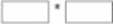## Which statement is TRUE concerning the "dropout" option?

Which statement is TRUE concerning the "dropout" option?
A . It specifies the number of neurons to drop from each layer of the network
B . It specifies the percentage of neurons to drop from a given layer of the network
C . It specifies the percentage of neurons to drop from the entire network
D . It specifies the number of layers to drop from the entire network

## Which statement is TRUE about a document that satisfies this rule?

Consider the category rule: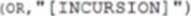Which statement is TRUE about a document that satisfies this rule?
A . It exhibits the topic named INCURSION
B . It exhibits one or more of the items used to define the INCURSION concept
C . It contains the term "incursion" or any of the stemmed versions of "incursion ‘
D . It satisfies a previously defined INCURSION category rule but overrides the primary Boolean operator in the original rule with the OR operator

## Which concept rule must be used for generating factual extraction within a sentence containing machine learning and text analytics?

Which concept rule must be used for generating factual extraction within a sentence containing machine learning and text analytics?

A)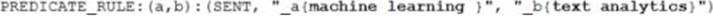B)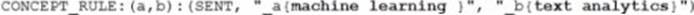C)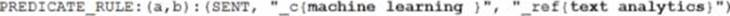D)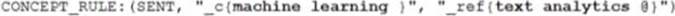A . Option A
B . Option B
C . Option C
D . Option D

## What are two reasons to use a pooling layer in a Convolution Neural Network (CNN)? (Choose two)

What are two reasons to use a pooling layer in a Convolution Neural Network (CNN)? (Choose two)
A . to increase granularity of information
B . to make the feature map invariant to transformation
C . to extract features of different spatial scale
D . to extract a summarized value from an area of the image

## What is the correct dimension of the output of this concatenation layer?

Given the code to add a concatenation layer: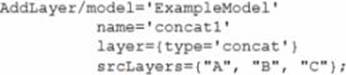Assume the output dimensions (width*depth):

• source layer A 100*100*5

• source layer B 100*100*5

• source layer C 100*100*3

What is the correct dimension of the output of this concatenation layer?
A . 100’100’13
B . 300’300’5
C . 100’100’18
D . 300’300’13

## What is the actual batch size?

Given the code to score images: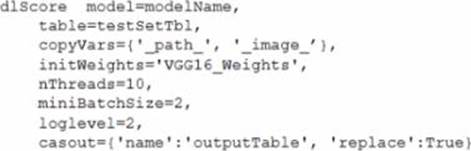What is the actual batch size?
A . 20
B . 2
C . 40
D . 10

## Which action set is needed to upload a picture into memory using SAS?

Which action set is needed to upload a picture into memory using SAS?
B . Image
C . DeepLearn
D . Table

## What is the depth of the residual layer depicted above’?

Refer to the exhibit.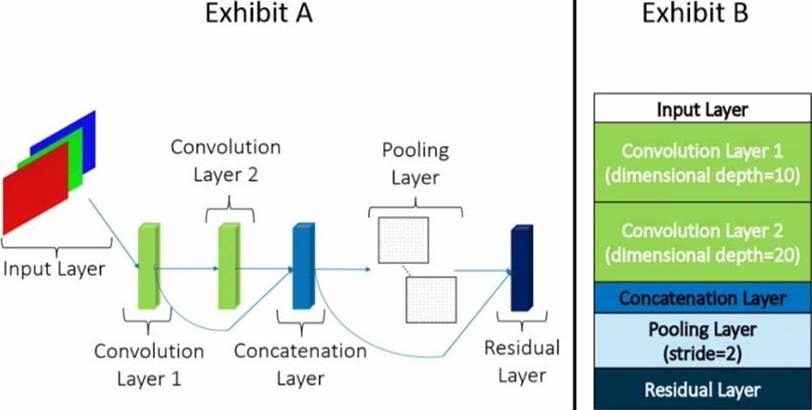Exhibit A details the structure of a convolutional model Exhibit B provides details o( each layer in the model The blue arrowed lines represent connections between layers.

What is the depth of the residual layer depicted above’?
A . 30
B . 60
C . 15
D . 45

## How many trainable parameters for layer fc2?

Given these two addLayer action calls: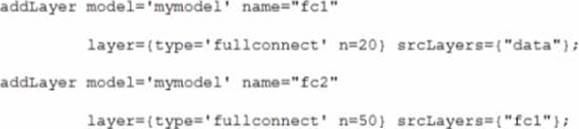How many trainable parameters for layer fc2?
A . 70
B . 1050
C . 1020
D . 1000

## What is the output feature map size created by the cross-correlation between a three color channel, 10*10 input and a 4"4 filter that has a stride of two?

CORRECT TEXT

Assume no padding is used for the input.

What is the output feature map size created by the cross-correlation between a three color channel, 10*10 input and a 4"4 filter that has a stride of two?